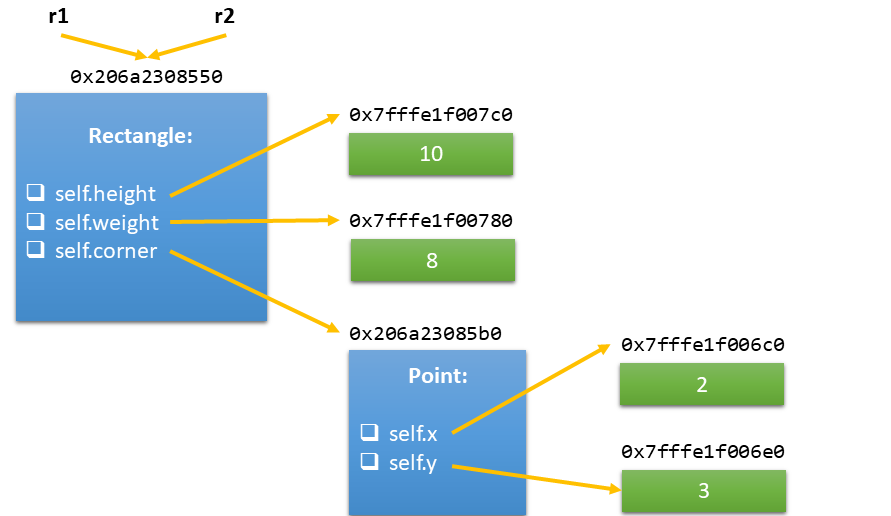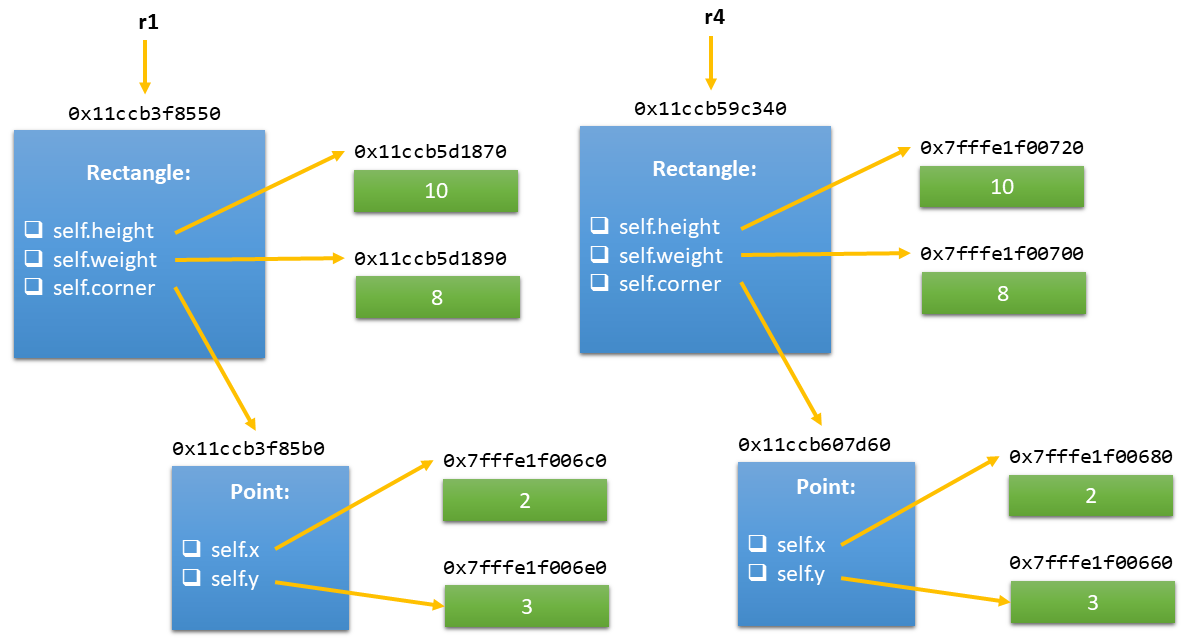Warning

# Computer Lab 11, objects and classes II

This week we will work with classes representing:

• 2D points: class Point (with attributes x (int), y (int) being coordinates of the point)
• Rectangles: class Rectangle (with attributes corner (type Point), width (int), height (int))

class Point:
"""Representation of a 2D point."""

def __init__(self, x, y):
"""Initializes the Point object.

Attributes:
x (float): x coordinate of the point.
y (float): y coordinate of the point.
"""
self.x = x
self.y = y

def move(self, dx, dy):
return Point(self.x + dx, self.y + dy)

def __str__(self):
return "Point(x={}, y={})".format(self.x, self.y)

def __eq__(self, other):
return (self.x == other.x and self.y == other.y)

class Rectangle:
"""Representation of a rectangle"""

def __init__(self, corner, height, width):
"""Initializes the Rectangle object.

Attributes:
corner (Point): coordinates of the left upper corner of the rectangle.
height (float): height of the rectangle.
width (float): width of the rectangle.
"""
self.corner = corner
self.height = height
self.width = width

def __str__(self):
return "Rectangle({}, h={}, w={})".format(str(self.corner), self.height, self.width)

def __eq__(self, other):
return (self.corner == other.corner and
self.height == other.height and
self.width == other.width)

#### Dot operator composition

If a function/method operating on an object is pure (returns a modified copy of the original object), it is common to use the dot operator multiple times.

if __name__ == "__main__":
p1 = Point(2, 3)
print(p1)

# dot operator composition
p2 = p1.move(1, -2)
print(p2)
p3 = p1.move(1, 0).move(0, -2)
print(p3)

#### Objects are mutable

Now, let's try changing the object's attributes via a (global) function:

def move_point(p, dx, dy):
p.x += dx
p.y += dy

    p1 = Point(2, 3)

# objects mutable
print(p1)
move_point(p1, 2, 3)
print(p1)

#### Object equality and identity

There are several ways to copy an object, each of which copies an object in a slight difference sense:

1. The = operator: Makes variables to point to the same object in memory
2. The copy.copy() function: Shallow copy (1 level deep). A shallow copy constructs a new compound object and then (to the extent possible) inserts references into it to the objects found in the original.
3. The copy.deepcopy() function: Deep copy (recursive). A deep copy constructs a new compound object and then, recursively, inserts copies into it of the objects found in the original.

There are also 2 ways of comparing 2 objects:

1. is and is not checks for identity: Everything in Python is an object, and each object is stored at a specific memory location. The Python is and is not operators check whether two variables refer to the same object in memory. Note: Keep in mind that objects with the same value are usually stored at separate memory addresses.
• == and != checks for equality: Checks whether or not two objects have the same value, regardless of where they’re stored in memory. In the vast majority of cases, this is what you want to do. The magic of the equality operator == happens in the __eq__() class method of the object to the left of the == sign. This is a magic class method that’s called whenever an instance of this class is compared against another object. If this method is not implemented, then == compares the memory addresses of the two objects by default (also see here). Check our implementation of __eq__() methods of the Point and Rectangle classes.

1. Copy using the “=” operator. The 2 variables pointing to the exact same object in memory.

if __name__ == "__main__":
r1 = Rectangle(Point(2, 3), 10, 8)
print("r1:", r1)

# copy using the "=" operator
# only reference is copied
# difference variables pointing to the exact same object
r2 = r1

print("r1 == r2: ", r1 == r2)
print("r1 is r2: ", r1 is r2)

print("\nr2.height = 5")
r2.height = 5
print("r1:", r1)
print("r2:", r2)
print("r1 == r2: ", r1 == r2)
print("r1 is r2: ", r1 is r2)

print("\nr2.corner.x = 0")
r2.corner.x = 0
print("r1:", r1)
print("r2:", r2)
print("r1 == r2: ", r1 == r2)
print("r1 is r2: ", r1 is r2)

r1: Rectangle(Point(x=2, y=3), h=10, w=8)
r1 == r2:  True
r1 is r2:  True

r2.height = 5
r1: Rectangle(Point(x=2, y=3), h=5, w=8)
r2: Rectangle(Point(x=2, y=3), h=5, w=8)
r1 == r2:  True
r1 is r2:  True

r2.corner.x = 0
r1: Rectangle(Point(x=0, y=3), h=5, w=8)
r2: Rectangle(Point(x=0, y=3), h=5, w=8)
r1 == r2:  True
r1 is r2:  True2. Copy using the copy.copy() fuction (shallow copy). Separate copies up to the 1st level.

if __name__ == "__main__":
r1 = Rectangle(Point(2, 3), 10, 8)
print("r1:", r1)

# copy using the copy.copy() function
# copy only 1 level deep
# called a shallow copy
r3 = copy.copy(r1)

print("r1 == r3: ", r1 == r3)
print("r1 is r3: ", r1 is r3)

print("\nr3.height = 5")
r3.height = 5
print("r1:", r1)
print("r3:", r3)
print("r1 == r3: ", r1 == r3)
print("r1 is r3: ", r1 is r3)

print("\nr3.corner.x = 0")
r3.corner.x = 0
print("r1:", r1)
print("r3:", r3)
print("r1 == r3: ", r1 == r3)
print("r1 is r3: ", r1 is r3)

r1: Rectangle(Point(x=2, y=3), h=10, w=8)
r1 == r3:  True
r1 is r3:  False

r3.height = 5
r1: Rectangle(Point(x=2, y=3), h=10, w=8)
r3: Rectangle(Point(x=2, y=3), h=5, w=8)
r1 == r3:  False
r1 is r3:  False

r3.corner.x = 0
r1: Rectangle(Point(x=0, y=3), h=10, w=8)
r3: Rectangle(Point(x=0, y=3), h=5, w=8)
r1 == r3:  False
r1 is r3:  False

3. Copy using the copy.deepcopy() function (deep copy). Separate copies including child objects.

if __name__ == "__main__":
r1 = Rectangle(Point(2, 3), 10, 8)
print("r1:", r1)

# copy using the copy.deepcopy() function
# copy of the child objects (recursively)
# called a deep copy
r4 = copy.deepcopy(r1)

print("r1 == r4: ", r1 == r4)
print("r1 is r4: ", r1 is r4)

print("\nr4.height = 5")
r4.height = 5
print("r1:", r1)
print("r4:", r4)
print("r1 == r4: ", r1 == r4)
print("r1 is r4: ", r1 is r4)

print("\nr4.corner.x = 0")
r4.corner.x = 0
print("r1:", r1)
print("r4:", r4)
print("r1 == r4: ", r1 == r4)
print("r1 is r4: ", r1 is r4)

r1: Rectangle(Point(x=2, y=3), h=10, w=8)
r1 == r4:  True
r1 is r4:  False

r4.height = 5
r1: Rectangle(Point(x=2, y=3), h=10, w=8)
r4: Rectangle(Point(x=2, y=3), h=5, w=8)
r1 == r4:  False
r1 is r4:  False

r4.corner.x = 0
r1: Rectangle(Point(x=2, y=3), h=10, w=8)
r4: Rectangle(Point(x=0, y=3), h=5, w=8)
r1 == r4:  False
r1 is r4:  False
Deep copyMotivation: The + operator will perform arithmetic addition on two numbers, merge two lists, or concatenate two strings. How should it work with your class?

• __str__(self): Controls how the object gets printed. Also, this same method is invoked when we use the built-in function str() or format().
• __eq__(self, other): Equality operator.
• __add__(self, other): The addition operation + is carried out the way we specify in this special method.
• __lt__(self, other): Implementation of the less than symbol < symbol in our class.
• Check all the arithmetic and comparison operators here.

class Point:
"""Representation of a 2D point."""

def __init__(self, x, y):
"""Initializes the Point object.

Attributes:
x (float): x coordinate of the point.
y (float): y coordinate of the point.
"""
self.x = x
self.y = y

return Point(self.x + other.x, self.y + other.y)

def __lt__(self, other):
norm_self_quadr = self.x ** 2 + self.y ** 2
norm_other_quadr = other.x ** 2 + other.y ** 2
return norm_self_quadr < norm_other_quadr

#### Weekly Homework 11 - Point object

Create a class representing a 2D point in polar coordinates. Each such point is characterized by an ordered pair $(r, \phi)$:

• $r$ - radial distance, distance from the origin to the point.
• $\phi$ - angular distance, the angle between the positive x-axis and the line segment between the origin and the point.

Your class must have the following structure. Copy-paste the following code and implement the (special) methods:

Note:

• Two points are equal if and only if both of their coordinates are the same.
• Assume: $p_1=(r_1, \phi_1)$, $p_2=(r_2, \phi_2)$
• Multiplication: $p_1 \cdot p_2 = (r_1 r_2, \phi_1 + \phi_2)$
• Division: $\frac{p_1}{p_2} = (\frac{r_1}{r_2}, \phi_1 - \phi_2)$
• The functions __mul__(self, other) and __truediv__(self, other) return an instance of the PointPolar class leaving both original points unchanged.
• Compare (<, ⇐, >, >=) two points by their radial distances only.
• In fact, it is possible for the angular distance to be over $2 \pi$ rad or less than $0$ rad, for example as a result of some operation. Please, assume that this won't happen with the supplied test cases in BRUTE. For the sake of simplicity, all input points and results of all operations will be guaranteed to have $\phi \in \left[ 0, 2 \pi \right]$ rad.

Required filename: 11_weekly_hw.py.

class PointPolar:
"""represents a point in polar coordinates"""

def __init__(self, r, phi):
"""
:param r: float, norm of the point
:param phi: float, angular coordinate
"""

def __str__(self):
"""
>>> p1 = PointPolar(5.1, 0.2)
>>> print(p1)
Point: r=5.1, phi=0.2
"""

def __eq__(self, other):
"""Equality given by both parameters"""

def __mul__(self, other):
"""Multiplication of 2 points"""

def __truediv__(self, other):
"""Division of 2 points"""

def __lt__(self, other):
"""Is self < other?"""

def __le__(self, other):
"""Is self <= other?"""

def __gt__(self, other):
"""Is self > other?"""

def __ge__(self, other):
"""Is self >= other?"""